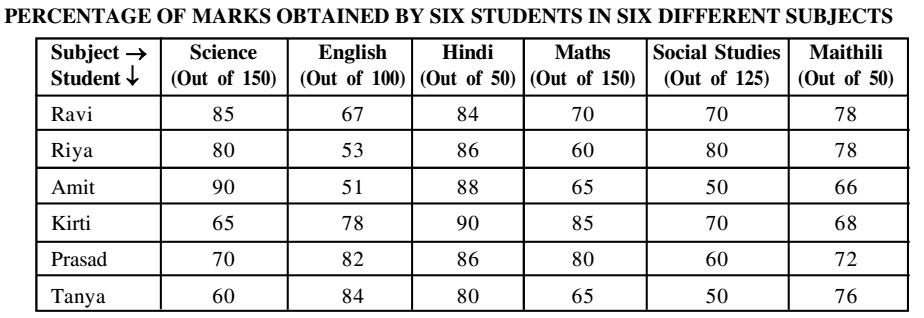Home » Data Interpretation » Table chart » Question

#### Data Interpretation

Direction: Study the table carefully to answer the questions that follow:1. What are the total marks obtained by Tanya in all the subject together ?
1. 402
2. 408
3. 412
4. 418
##### Correct Option: C

Total marks obtained by Tanya in all the subjects
= 150 x 60% + 84 + 50 x 80% + 150 x 65% + 125 x 50 + 50 x 76%
Total marks obtained by Tanya in all the subjects
= 150 x 60 /100 + 84 + 50 x 80/100 + 150 x 65/100 + 125 x 50/100 + 50 x 76/100
= 90 + 84 + 40 + 97.5 + 62.5 + 38 = 412